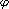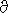# Thermal Wind Equation

Relationship between meridional temperature gradient and vertical wind shear due to non-uniform horizontal heating. Note: dy = Re · d. Hydrostatic and geostrophic balance yields:Ug/P = (1/f)/y (1/) = Rg/(f · P) (dT/dy)
U(z2) = U(z1) - (R/f) · dT/dy|P · log(P1/P2)
f0 dU/dz = (Rg/H) (dT/dy)